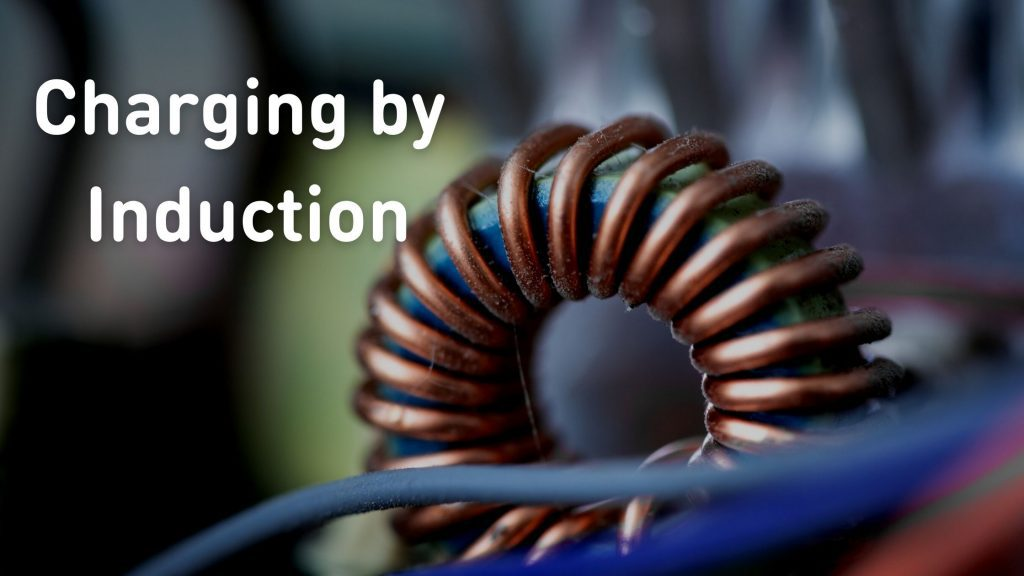Physics
Study Material

# Charging By Induction

Most objects are electrically neutral, which means that they have an equal number of positive and negative charges. In order to charge an object, one has to alter the charge balance of positive and negative charges. There are three ways to do it: friction, conduction and induction.

2 minutes long
Posted by Mahak Jain, 1/12/2021Hesap Oluştur

Got stuck on homework? Get your step-by-step solutions from real tutors in minutes! 24/7. Unlimited.

Most objects are electrically neutral, which means that they have an equal number of positive and negative charges. In order to charge an object, one has to alter the charge balance of positive and negative charges. There are three ways to do it: friction, conduction and charging by induction.

## Charging by Friction:

The charging by friction process involves rubbing of one particle on another resulting in electrons moving from one surface to another. This method is useful for charging insulators.

## Charging by Conduction:

The charging by conduction process involves touching of a charged particle to a conductive material. This way, the charges are transferred from the charged material to the conductor. This method is useful for charging conductors.

## Charging By Induction:

Lastly, the induction charging is a charging method that charges an object without actually touching the object to any another charged object. The charging by induction process is where the charged particle is held near an uncharged conductive material that is grounded on a neutrally charged material. The charge flows between two objects and the uncharged conductive material develop a charge with opposite polarity.

These were the different methods of charge transfer. Here, in the article let us learn more about the method of charging by induction.

## Charging by induction using a negatively charged object

In this section, we shall learn about the transfer of charge by induction using a negatively charged object. Let us consider two metal spheres A and B touching each other, as shown in the figure. Let us take a negatively charged rubber balloon. If we bring the charged balloon near the spheres, electrons within the two-sphere system will be induced to move away from the balloon due to the repulsion between the electrons of the balloon and the spheres. Subsequently, the electrons from sphere A get transferred to sphere B.

The migration of electrons causes the sphere A to become positively charged and the sphere B to be negatively charged. The overall two-sphere system is hence electrically neutral. The spheres are then separated using an insulating cover such as gloves or a stand as shown in the figure (avoiding direct contact with the metal). When we remove the balloon, the charge gets redistributed, spreading throughout the spheres, as shown in the figure.